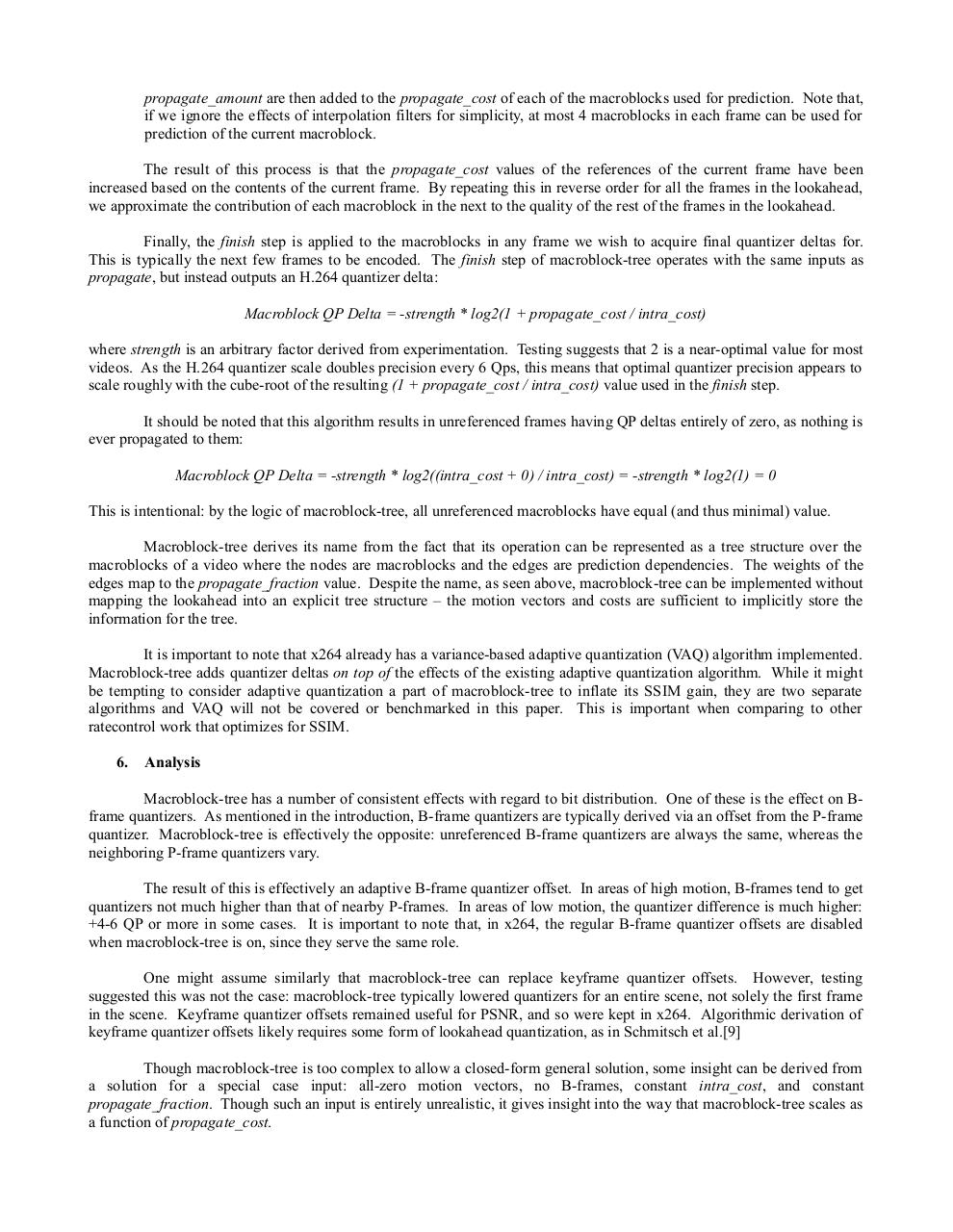# MBtree paper.pdfPage 1 2 3 4 5 6 7 8 9 10 11 12

#### Text preview

propagate_amount are then added to the propagate_cost of each of the macroblocks used for prediction. Note that,
if we ignore the effects of interpolation filters for simplicity, at most 4 macroblocks in each frame can be used for
prediction of the current macroblock.
The result of this process is that the propagate_cost values of the references of the current frame have been
increased based on the contents of the current frame. By repeating this in reverse order for all the frames in the lookahead,
we approximate the contribution of each macroblock in the next to the quality of the rest of the frames in the lookahead.
Finally, the finish step is applied to the macroblocks in any frame we wish to acquire final quantizer deltas for.
This is typically the next few frames to be encoded. The finish step of macroblock-tree operates with the same inputs as
propagate, but instead outputs an H.264 quantizer delta:
Macroblock QP Delta = -strength * log2(1 + propagate_cost / intra_cost)
where strength is an arbitrary factor derived from experimentation. Testing suggests that 2 is a near-optimal value for most
videos. As the H.264 quantizer scale doubles precision every 6 Qps, this means that optimal quantizer precision appears to
scale roughly with the cube-root of the resulting (1 + propagate_cost / intra_cost) value used in the finish step.
It should be noted that this algorithm results in unreferenced frames having QP deltas entirely of zero, as nothing is
ever propagated to them:
Macroblock QP Delta = -strength * log2((intra_cost + 0) / intra_cost) = -strength * log2(1) = 0
This is intentional: by the logic of macroblock-tree, all unreferenced macroblocks have equal (and thus minimal) value.
Macroblock-tree derives its name from the fact that its operation can be represented as a tree structure over the
macroblocks of a video where the nodes are macroblocks and the edges are prediction dependencies. The weights of the
edges map to the propagate_fraction value. Despite the name, as seen above, macroblock-tree can be implemented without
mapping the lookahead into an explicit tree structure – the motion vectors and costs are sufficient to implicitly store the
information for the tree.
It is important to note that x264 already has a variance-based adaptive quantization (VAQ) algorithm implemented.
Macroblock-tree adds quantizer deltas on top of the effects of the existing adaptive quantization algorithm. While it might
be tempting to consider adaptive quantization a part of macroblock-tree to inflate its SSIM gain, they are two separate
algorithms and VAQ will not be covered or benchmarked in this paper. This is important when comparing to other
ratecontrol work that optimizes for SSIM.
6.

Analysis

Macroblock-tree has a number of consistent effects with regard to bit distribution. One of these is the effect on Bframe quantizers. As mentioned in the introduction, B-frame quantizers are typically derived via an offset from the P-frame
quantizer. Macroblock-tree is effectively the opposite: unreferenced B-frame quantizers are always the same, whereas the
neighboring P-frame quantizers vary.
The result of this is effectively an adaptive B-frame quantizer offset. In areas of high motion, B-frames tend to get
quantizers not much higher than that of nearby P-frames. In areas of low motion, the quantizer difference is much higher:
+4-6 QP or more in some cases. It is important to note that, in x264, the regular B-frame quantizer offsets are disabled
when macroblock-tree is on, since they serve the same role.
One might assume similarly that macroblock-tree can replace keyframe quantizer offsets. However, testing
suggested this was not the case: macroblock-tree typically lowered quantizers for an entire scene, not solely the first frame
in the scene. Keyframe quantizer offsets remained useful for PSNR, and so were kept in x264. Algorithmic derivation of
keyframe quantizer offsets likely requires some form of lookahead quantization, as in Schmitsch et al.
Though macroblock-tree is too complex to allow a closed-form general solution, some insight can be derived from
a solution for a special case input: all-zero motion vectors, no B-frames, constant intra_cost, and constant
propagate_fraction. Though such an input is entirely unrealistic, it gives insight into the way that macroblock-tree scales as
a function of propagate_cost.